## Table of ContentOpen Access

ARTICLE

# Two-Sided Matching Decision Making with Multi-Attribute Probabilistic Hesitant Fuzzy Sets

Peichen Zhao1, Qi Yue2,*, Zhibin Deng3

1 School of Mathematics and Statistics, Heze University, Heze, 274015, China
2 School of Management, Shanghai University of Engineering Science, Shanghai, 201620, China
3 School of Information Management, Jiangxi University of Finance and Economics, Nanchang, 330013, China

* Corresponding Author: Qi Yue. Email:Intelligent Automation & Soft Computing 2023, 37(1), 849-873. https://doi.org/10.32604/iasc.2023.037090

## Abstract

In previous research on two-sided matching (TSM) decision, agents’ preferences were often given in the form of exact values of ordinal numbers and linguistic phrase term sets. Nowdays, the matching agent cannot perform the exact evaluation in the TSM situations due to the great fuzziness of human thought and the complexity of reality. Probability hesitant fuzzy sets, however, have grown in popularity due to their advantages in communicating complex information. Therefore, this paper develops a TSM decision-making approach with multi-attribute probability hesitant fuzzy sets and unknown attribute weight information. The agent attribute weight vector should be obtained by using the maximum deviation method and Hamming distance. The probabilistic hesitancy fuzzy information matrix of each agent is then arranged to determine the comprehensive evaluation of two matching agent sets. The agent satisfaction degree is calculated using the technique for order preference by similarity to ideal solution (TOPSIS). Additionally, the multi-object programming technique is used to establish a TSM method with the objective of maximizing the agent satisfaction of two-sided agents, and the matching schemes are then established by solving the built model. The study concludes by providing a real-world supply-demand scenario to illustrate the effectiveness of the proposed method. The proposed method is more flexible than prior research since it expresses evaluation information using probability hesitating fuzzy sets and can be used in scenarios when attribute weight information is unclear.

## Keywords

1  Introduction

Two-sided matching decision-making (TSMDM) consists of two sides of matching agents and belongs to the decision-making problems , which was first proposed and applied by Gale and Shapley in a study of matchmaking and college admission . The agent, also called as the intermediary, is what determines the result of the matching process but does not actually participate in the matching process directly. The matching agents could play various roles in various scenarios requiring decision-making . If you search or buy a product online today, you will find more of the same products on the website tomorrow; this indicates that the matching system has automatically matched you as a potential customer. Both sides of matching agents participate in the matching process, and using a given matching technique, all matching agents are evaluated by those on the other side. The formation of a perfect matching scheme which is satisfied by all matching agents must be facilitated by the intermediary based on the preferences or evaluations provided by the matching agent.

TSMDM is now used in a wide range of fields, such as marriage matching problems [4,5], colleges admissions , volunteer team dispatching , human resource management , economic management matching , public-private partnership , and knowledge service matching . The two-sided matching theory has been used in a variety of areas recently, along with the development of the market economy and the level of science and technology. For instance, Wu et al.  studied the supply-demand issue in transport services and developed the corresponding TSMDM method. Wang et al.  has developed a novel matching method for solving the freight source matching problem. Liu et al. proposed a unique TSMDM method with the objective of achieving satisfying matching between surgeons and patients . For the purpose of resolving the existing house seller-buyer matching problem, Jiang et al.  proposed a novel TSMDM.

Meantime, a significant number of scholars have studied the multi-attribute TSMDM problem. For instance, Jiang et al.  proposed a multi-objective non-linear model to address one-shot multi-attribute exchange problems. Based on the Choquet integral aggregation operator, a novel TSMDM method was developed by Liang et al. , which was used to solve the multi-attribute TSMDM problems considering q-Rung Orthopair fuzzy information. Liang et al.  developed a novel TSMDM model based on the strict order number information. In light of multi-granularity probabilistic linguistic MARCOS, Lu et al.  proposed a multi-attribute two-sided matching method.

Some researchers studied the TSMDM problem with complete or partial preference ordering in the previous studies. For instance, Pu et al.  proposed a novel TSMDM method under the goal of maximizing intermediary revenue and overall agent satisfaction. Fan et al.  studied the TSMDM problem with uncertain preference ordinal and proposed the corresponding TSMDM model. A novel TSMDM method was developed by Chen et al.  after studying the TSMDM problem in perspective of psychological behaviors. Qin et al.  proposed an optimization method for resolving the TSMDM problem with incomplete weak preference ordering. To express the evaluation information in the practical TSMDM problem, linguistic evaluation was also applied. For instance, Lin et al.  studied the multi-attribute TSMDM problems with 2-tuple linguistic information and proposed a new TSMDM method. Considering the multi-granular HFLTSs environment, Zhang et al.  developed a comprehensive TSMDM method considering the stable one-to-one matching constraints.

Researches conducted by many scholars have provided sufficient solutions for the two-sided matching problem under different scenarios. However, decision-makers find it difficult to deliver precise and concise information given the complexity of the real world. In certain situations, decision-makers are unable to convey the whole significance of the evaluation information. To describe the evaluation information, several fuzzy information express tools would be employed, such as interval numbers, fuzzy numbers, hesitant fuzzy sets, intuitionistic fuzzy sets  and others.

PHFS, which is an important extended form of the hesitant fuzzy set and a close and effective combination of membership degree and probability distribution information, was first proposed by Zhu . Probabilistic hesitating fuzzy sets, as compared to HFS, are more capable of dealing with and explain the information of preference evaluation in complex decision-making situations. In real world, significant and vital decisions are usually made by the group which consists of multiple members. Due to the inaccurate estimations and lack of expertise, the evaluation information provided by experts may have different assessment values. Therefore, the information of the probabilistic hesitant fuzzy element (PHFE) may be used to express the overall opinion formed by these different evaluation results. For instance, suppose a company invites ten experts to evaluate a scheme (score between [0, 1]), and let’s say that six of the ten experts give the scheme a score of 0.5 and four experts give it a score of 0.7. The evaluation information can be provided by the PHFE {0.5|0.6,0.7|0.4}. The PHFS theory can be applied to handle imperfect, vague, and imprecise information. Thus PHFS has been widely applied in economics , emergency management [29,30], and decision-making . Meanwhile, some theoretical foundations have been established, such as aggregation operations , distance measures , and entropy measures . Because of the major difference in individual cognition and the lack of knowledge structure, the two-sided agents are used to express the evaluation information by PHFS. Based on this overview of previous literature, the probability hesitant fuzzy environment in multi-attribute TSMDM problems has not been given much attention. Therefore, a TSMDM method, considering the PHFS with incomplete attribute weight information, will be proposed in this paper. By using the TOPSIS method, the solution strategy is developed and the matching result takes into account the maximum degree of satisfaction on both sides of the matching agents. Additionally, a supply-demand example for catering services will be provided to highlight the usefulness and viability of the proposed model. The new aspects of this paper are: (1) the development of a novel two-sided matching decision-making method in a probability hesitant fuzzy environment; and (2) the application of the proposed method to the two-sided matching problem with incomplete attribute weight.

The rest of this paper is organized as follows. Some preliminaries about TSMDM and PHFS will be presented in Section 2. The proposed problem is explained in Section 3, and builds the framework for the proposed method. In Section 4, a case is provided to demonstrate the effectiveness of the proposed method. Additionally, Section 4 explains the characteristics of the proposed TSMDM method. Finally, the main contributions of the proposed method are presented in Section 5.

2  Preliminaries

The concept of two-sided matching is given in this section, and then some related theories of PHFSs are introduced, including the concept, operation rules, score function and aggregation operation. Finally, it discusses the distance measure of PHFSs and TOPSIS method.

2.1 Two-Sided Matching

Two matching agent sets, A and B, are given in this subsection. Let A={A1,A2,,Am} denote the set of m matching objects, where Ai denotes the ith agent in A. Let B={B1,B2,,Bn} be the set of n matching objects, where Bj denotes the jth agent in B, and thus satisfies nm2, M={1,,m},N={1,,n}.

Definition 1 . Suppose μ: ABAB is a one-to-one map, AiA, BjB, satisfying: i) μ(Ai)B; ii) μ(Bj)A{Bj}; iii) μ(Ai)=Bj if and only if μ(Bj)=Ai.

Then, μ refers to the matching scheme between set A and set B, where μ(Ai)=Bj denotes that Ai is matched with Bj in μ and the (Ai,Bj) is defined as a matching pair, μ(Ai)Bk denotes that Ai is not matched with Bk in μ. Specially, when μ(Bj)=Bj, then Bj is unmatched in μ. As nm2, each matching object in A must be matched with one matching object in B and nm matching objects in B are unmatched.

2.2 Probabilistic Hesitant Fuzzy Sets

HFS was first proposed by Torra  and further expressed in mathematical symbol by Xia et al. . Unlike other extensions of the fuzzy set, HFS allows the membership degree of an element to a set presented by several possible values, which can express the uncertain information more comprehensively. However, the probability of each membership degree is the same. To better represent the preference information, by incorporating probability to describe the preference information in HFS, Zhu  proposed the concept of PHFS. Compared with HFSs, the following PHFSs can reserve more original information.

Definition 2 [27,32]. Let X be a fixed set. Then, a PHFS on X is H={<x,h(γi(x)|pi(x))>|xX}, where, h(γi(x)|pi(x)) is a set of elements and γi(x)|pi(x) is a subset of [0,1] denoting the hesitant fuzzy information with probabilities to the set H. For convenience, h(γi(x)|pi(x)) is called a probabilistic hesitant fuzzy element (PHFE), and PHFE h(γi(x)|pi(x)) is denoted by h(p), then h(p)={γi|pi,i=1,2,,#h}, where pi is the probability of the membership degree γi and i=1#hpi=1,γi|pi is considered as a term of the PHFE, #h is the total number of different membership degrees. In this paper, we arrange the terms γi|pi in increasing order based on the value of γi.

Remark 1: In Definition 2, i=1#hpi=1 states that the PHFE includes the complete probabilistic information. i=1#hpi<1 states that the probability information is incomplete. Therefore, the following formula can be used to transform the PHFE with incomplete probability information into the PHFE with complete probability information: pi¯=pii=1#hpi,i=1,,#h. For decision makers with different culture and knowledge backgrounds, it is possible that i=1#hpi<1. In this paper, we assume that i=1#hpi1.

Remark 2: In Definition 2, the PHFE h(p)={γi|pi,i=1,2,,#h} of PHFS may be revised according to the multiplier of the membership degree γi and the corresponding probability pi, and then re-order the terms γipi in increasing order. For instance (0.5|0.4,0.6|0.2,0.7|0.4) can be revised as (0.12,0.2,0.28).

Definition 3 [27,32]: Let h(p),h1(p1),h2(p2) be three PHFEs and α>0, then some operation rules among them are defined as:

i)   (h(p))α=γh(p){γα|p};

ii)   αh(p)=γh(p){1(1γ)α|p};

iii)   (h(p))c=γh(p){1γ|p};

iv)   h1(p1)h2(p2)==γ1h1(p1),γ2h2(p2){1(1γ1)(1γ2)|p1p2};

v)   h1(p1)h2(p2)=γ1h1(p1),γ2h2(p2){γ1γ2|p1p2}.

Definition 4 [27,32]: Let h(p)={γi|pi,i=1,2,,#h} be a PHFE, a score function is defined by:

S(h(p))=i=1#hγipi(1)

According to the score function, the deviation function is defined by:

V(h(p))=i=1#h(γiS(h(p)))2pi(2)

According to the score functions, we can compare PHFEs h1(p) and h2(p) based on the following rules:

if S(h1)>S(h2), then h1(p)h2(p);

if S(h1)<S(h2), then h1(p)h2(p);

if S(h1)=S(h2): if V(h1)>V(h2), then h1(p)h2(p); if V(h1)<V(h2), then h1(p)h2(p);if V(h1)=V(h2), then h1(p)h2(p).

2.3 Aggregation Operators and Distance Measures for PHFEs

Zhang et al.  proposed the probabilistic hesitant fuzzy weighted averaging (PHFWA) operator and the probabilistic hesitant fuzzy weighted geometric (PHFWG) operator, both of which are very useful in decision-making using probabilistic hesitant fuzzy information.

Definition 5 . Let h1(p1),h2(p2)hn(pn) be PHFEs, and wj(j=1nwj=1) be the weight of PHFEs hj(pj), then a probabilistic hesitant fuzzy weighted averaging (PHFWA) operator and a probabilistic hesitant fuzzy weighted geometric (PHFWG) operator are stated as follows:

PHFWA(h1(p1),h2(p2)hn(pn))=j=1nwjhj(pj)=γjhj(pj){1j=1n(1γj)wj|p1pn}(3)

PHFWG(h1(p1),h2(p2)hn(pn))=j=1nhj(pj)wj=γjhj(pj){j=1nγjwj|p1pn}(4)

These two operators can transform the fuzzy decision information of the decision makers into aggregated decision information to identify the best option out of all feasible choices.

The definitions of the Hamming distance and the Euclidean distance of PHFEs are given as follows:

Definition 6 . Let h1(p1) and h2(p2) be two PHFEs. If #h=#h2=#h1, the number of elements in h1(p1) and h2(p2) are the same, then the normalized probabilistic hesitant fuzzy Hamming distance measure between h1(p1) and h2(p2) is defined as dH(h1,h2), and the normalized probabilistic hesitant fuzzy Euclidean distance measure between h1(p1) and h2(p2) is defined as dE(h1,h2),

dH(h1,h2)=i=1#h|h1σ(i)(p1σ(i))h2σ(i)(p2σ(i))|=i=1#h|γ1σ(i)p1σ(i)γ2σ(i)p2σ(i)|(5)

dE(h1,h2)=1#hi=1#h|h1σ(i)h2σ(i)|2=1#hi=1#h|γ1σ(i)p1σ(i)γσ(i)2p2σ(i)|2(6)

where γ1σ(i) and γ2σ(i) are the ith biggest values in h1(p1) and h2(p2).

Remark3: In most cases, #h2#h1. For the sake of calculation convenience, we need to treat the small-length probabilistic hesitancy fuzzy elements with equal length. The short-length probability hesitancy fuzzy element is supplemented by a smaller or larger membership degree element depending on the decision-makers’ various risk preferences. If the decision maker is risk-averse, add the element with the lowest value; if the decision maker likes taking risk, add the element with the highest value; if the decision maker is risk-neutral, add the average of the maximum and minimum values in the set. Let the probability of adding elements be 0, so that the correlation calculation remains unchanged even after adding elements. In this paper, the decision makers are assumed to be pessimists who expect unfavorable outcomes and may add the minimum value, and we define that the probability of each added value is 0. For example, h1(p1) and h2(p2) are two PHFEs, let h1(p1)=(0.5|0.5,0.7|0.5), h2(p2)=(0.5|0.3,0.6|0.3,0.7|0.4). Apparently, #h2>#h1; then, we extend h1(p1) until it has the same length as h2(p2), that is h1(p1)=(0.5|0,0.5|0.5,0.7|0.5). According to the Hamming distance measure, dH(h1,h2) can be calculated as:

dH(h1,h2)=|0.5×00.3×0.5|+|0.5×0.50.6×0.3|+|0.7×0.50.7×0.4|=0.15+0.07+0.07=0.29.

Definition 7 . The probabilistic hesitant fuzzy Positive Ideal Solution (PIS) is given as: h+(p)={1|1#h,1|1#h,,1|1#h}, and the probabilistic hesitant fuzzy Negative Ideal Solution (NIS) is given as: h(p)={0|1#h,0|1#h,,0|1#h}, respectively.

2.4 The TOPSIS Method for PHFS

The TOPSIS method, which was first proposed by Hwang and Yoon, is essential for processing information from multi-attribute evaluations and is a topic of much scholarly discussion . According to the PHFSs Hamming distance measure and the basic idea of traditional TOPSIS method, let D be the distance measure from the NIS and D+ be the distance measure from the PIS. Obviously, the larger the distance D+ the better the alternative, while the smaller the distance D the better the alternative . The alternative is defined as follows in order to represent the alternative closeness coefficient:

C=DD++D(7)

The closeness coefficients C for each alternative are calculated for both, the distance D+ and the distanceD simultaneously. The optimal solution is the one that comes closest to the positive ideal solution and is farthest from the negative ideal solution. From the above analysis, we have come to know that the higher the closeness coefficient, the better the alternative.

3  The Approach of TSMDM with PHFSs Information

In this section, the TSMDM problem with PHFSs is introduced and a novel TSMDM method is proposed.

3.1 Problems Description

Two agent sets are given in the two-sided matching problem which is addressed in this paper. Considering the fuzziness of the thinking of the matching subject and the disparity in knowledge organization, the evaluation information given by the two-sided agent is presented in the form of PHFS, and the attribute weight information given is also incomplete. The problem studied in this paper is to promote the formation of equitable bilateral matching results based on the information of probabilistic hesitancy fuzzy sets given by two-sided agents. The main notations used in this section are presented as follows:

A={A1,A2,,Am}: The set of matching objects on the side A, Ai(i=1,,m) denotes the ith matching object in A.

B={B1,B2,,Bn}: The set of matching objects on the side B, Bj denotes the j th matching object in B.

CA={C1A,C2A,,CsA}: The set of attributes used by matching objects A when providing PHFE assessments over matching objects B, where CkA(k=1,,s) is the kth attribute that the matching objects A pay attention to.

CB={C1B,C2B,,CtB}: The set of attributes used by matching objects B when providing PHFE assessments over matching objects A, where ClB(l=1,,t) is the lth attribute that the matching objects pay attention to.

wAi={w1Ai,w2Ai,,wsAi}: The weight vector of the attribute used by Ai, where wkAi is the weight of the kth attribute in CA, wkAi>0,k=1,2s and k=1swkAi=1, iM.

wBj={w1Bj,w2Bj,,wtBj}: The weight vector of the attributes used is the weight of the lth attribute in CB, wlbj>0,l=1,2t and l=1twlBj=1,jN.

HAi=(hijkA)n×s: The matrix about matching objects B provided by Ai, where hijkA is Ai’s PHFE assessment over Bj with respect to CkA.

HBj=(hijlB)m×t: The evaluation matrix from objects to Bj, where hijlB is Bj’s PHFE assessment over Ai with respect to ClB.

For the matching object Ai, let hijkA denote his/her assessment for the matching object Bj in accordance with the attribute CkA. The kth attribute’s weight in CA is due to wkAi, then Ai’s overall assessments of Bj can be obtained by hijA=k=1shijkAwkAi, all these HPFEs are contained in the probabilistic hesitant fuzzy matrixes HAi=(hijkA)n×s(i=1,m). For the matching object Bj, let hijlB denote his/her assessment for the matching object Ai with respect to the attribute ClB. Owing to wlBj is the weight of the lth attribute in CB, then Bj’s overall assessments of Ai can be obtained by hijB=l=1thijlBwlBj, all these PHFEs are contained in the probabilistic hesitant fuzzy matrixes HBj=(hijlB)m×t(j=1,n).

3.2 The Maximizing Deviation Method to Obtain the Weights of Attribute

Weight plays an essential role in reaching a reasonable decision result. The matching subject is unable to provide a comprehensive attribute weight vector due to the diversity and fuzziness of the information in the real decision-making environment. At the same time, Wang  puts forward a maximum deviation method to determine the attribute weight, and the core idea is to give the attribute weight based on the evaluation information given by the decision-maker. Some models will be proposed to determine the attribute weight vector for a two-sided agent based on the maximum deviation method.

In relation to the kth attribute CkA in CA, if matching object Ai provides assessment to the matching object Bj and Bf, denoted by hijkA and hifkA, then Definition 6 states that:

dH(hijkA,hifkA)=y=1#h|hjσ(y)(pjσ(y))hfσ(y)(pfσ(y))|=y=1#h|γjσ(y)pjσ(y)γfσ(y)pfσ(y)|(8)

The total deviation among Ai’s PHFE assessments over B can be calculated by:

dH(Aik)=j=1nf=1ndH(hijkA,hifkA)(9)

We can evaluate the total variance between Ai’s PHFE assessments over B with regard to all attributes by considering the weights of each attribute.

dH(Ai)=k=1swkAidH(Aik)=k=1sj=1nf=1nwkAidH(hijkA,hifkA)(10)

Then, a constrained condition k=1s(wkAi)2=1 for the weight vector wAi={w1Ai,w2Ai,,wsAi} is introduced based on Wang’s analysis. On this basis, the optimal weight vector is determined.

Therefore, a model for computing the attribute weight for Ai can be presented as:

maxk=1sj=1nf=1nwkAidH(hijkA,hifkA)s.t.k=1s(wkAi)2=1wkAi0,k=1,,s(11)

By using the Lagrange multiplier method and normalizing the weight vector, we have

wkAi=j=1nf=1ny=1#h|γjσ(y)pjσ(y)γfσ(y)pfσ(y)|k=1sj=1nf=1ny=1#h|γjσ(y)pjσ(y)γfσ(y)pfσ(y)|(k=1,,s)(12)

Similar to that, the attribute weight vector for Bj, i.e., wBj={w1Bj,w2Bj,,wtBj} can be calculated as:

wlBj=i=1mu=1mz=1#h|γiσ(z)piσ(z)γuσ(z)puσ(z)|l=1ti=1mu=1mz=1#h|γiσ(z)piσ(z)γuσ(z)puσ(z)|(l=1,,t)(13)

Furthermore, the matrices HA=(hijA)m×n and HB=(hijB)m×n can be calculated by aggregating the evaluation values based on the PHFWA operator.

3.3 The Satisfaction Degrees

First, the Hamming distance measure between the comprehensive evaluation of each matching agent and their corresponding positive and negative ideal evaluation is calculated, that is:

daij+=dH(hijA,hijA+),daij=dH(hijA,hijA),dbij+=dH(hijB,hijB+),dbij=dH(hijB,hijB)(14)

Then, the closeness coefficients of each matching agent could be computed by:

φaij=daijdaij++daij,φbij=dbijdbij++dbij(15)

The degree of divergence within the matching agent sets could clearly be observed in the closeness coefficients of each matching. For the matching agent Ai to the matching agent Bj, the small the distance daij+ and the larger the distance daij, the higher the closeness coefficient φaij. For the matching agent Bj to the matching agent Ai, the small the distance dbij+ and the larger the distance dbij, the higher the closeness coefficient φbij. The higher the closeness coefficient φaij meaning the higher the satisfaction of the matching agent Ai to the matching agent Bj; the higher the closeness coefficient φbij meaning the higher the satisfaction of the matching agent Bj to the matching agent Ai. From the above analysis, we can find that the closeness coefficient information can be utilized to measure the satisfaction degree. Therefore, we can establish the satisfaction degree matrices SA=(φaij)mn and SB=(φbij)mn to represent the satisfaction degree of the matching agent Ai to the matching agent Bj, and the satisfaction degree of the matching agent Bj to the matching agent Ai, respectively.

3.4 The TSMDM Model for PHFS

Additionally, a TSMDM model could be developed to obtain the best matching. Suppose xij represents a binary variable, when xij=0; it implies that Ai is not matched with Bj; xij=1 implies that Ai is matched with Bj and thus we have a matching pair (Ai,Bj). Consequently, a multi-objective programming model can be constructed as follows based on thorough consideration of agent satisfaction maximization:

maxZA=i=1mj=1nφaijxij(a)maxZB=i=1mj=1nφbijxij(b)s.t.i=1mxij1,j=1,2,n(c)j=1nxij1,i=1,2,m(d)xij={0,1},i=1,2,m;j=1,2,n(e)(16)

In model (16), formula (a) and formula (b) aim to maximize the overall satisfaction. And formula (c) and formula (d) refer to the one-to-one matching constrains.

3.5 Model Solution

We apply the linear weighted method to solve the multi-objective optimization model, and thus model (17) could be constructed as follows:

maxZ=wAi=1mj=1nφaijxij+wBi=1mj=1nφbijxij=i=1mj=1nφijxijs.t.i=1mxij1,j=1,2,nj=1nxij1,i=1,2,mxij={0,1},i=1,2,m;j=1,2,n(17)

where φij=wAφaij+wBφbij; wA and wB refer to the different importance of two-sided agent sets, which satisfies wA,wB(0,1),wA+wB=1. For both sides in the actual TSMDM, the goal ZA and ZB have the same status, we can allow wA=wB, if the goal ZA is more important than ZB. We can also let wA>wB. Finally, we can use Matlab or Lingo 11 to solve model (17).

3.6 The Steps

In conclusion, the steps of the proposed TSMDM approach can be summed up as follows (see Fig. 1 below):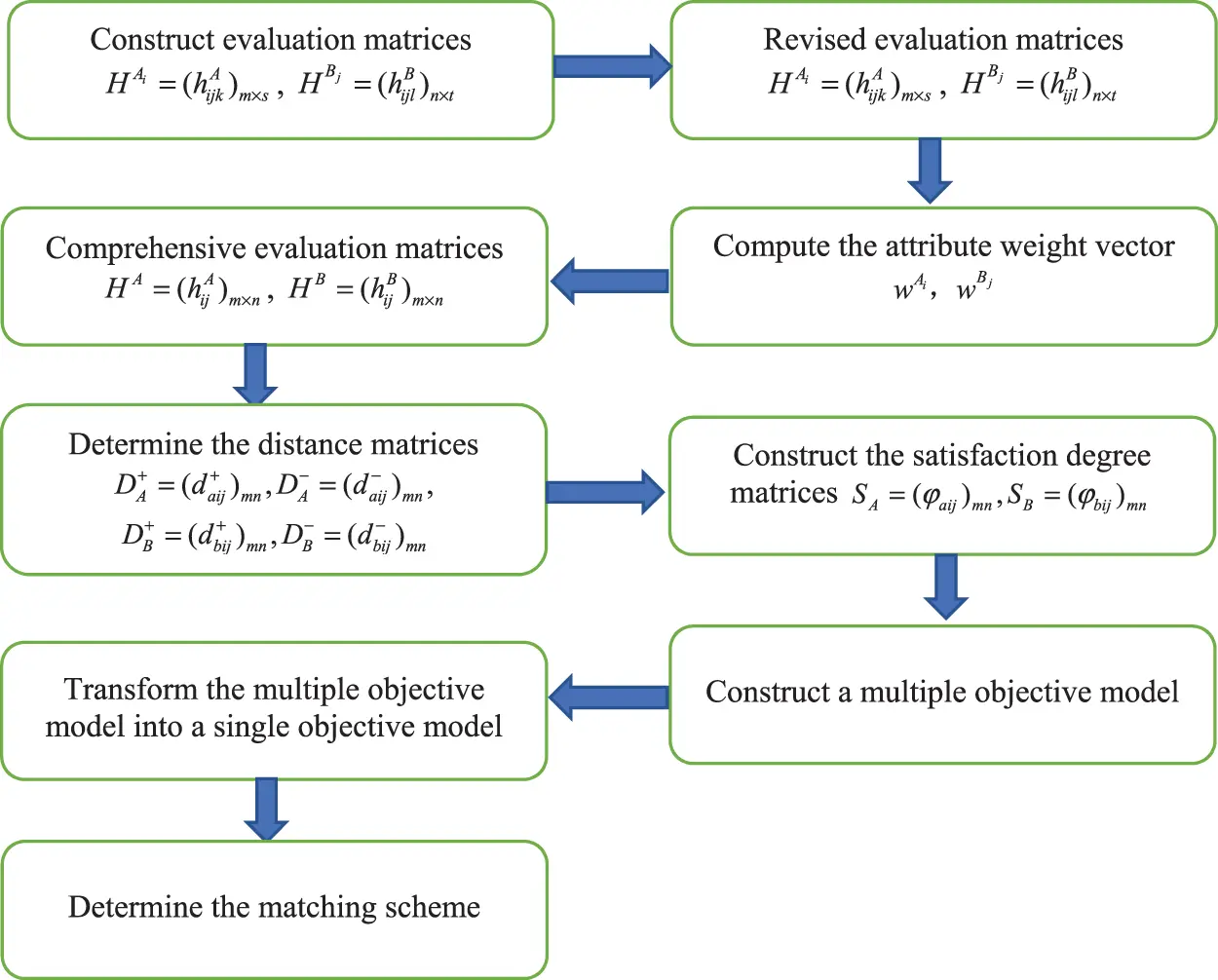Figure 1: The procedure of two-sided matchingStep 1: Construct evaluation matrices HAi=(hijkA)m×s(i=1,m) and HBj=(hijlB)n×t(j=1,n) based on the PHFEs information given by two-sided agents.

Step 2: Compute the attribute weight vector wAi={w1Ai,w2Ai,,wsAi} and wBj={w1Bj,w2Bj,,wtBj}according to Eqs. (12) and (13).

Step 3: According to the Eq. (3), transform evaluation matrices HAi=(hijkA)m×s (i=1,m) and HBj=(hijlB)n×t(j=1,n) into evaluation matrices HA=(hijA)m×n and HB=(hijB)m×n based on the attribute weight vector wAi={w1Ai,w2Ai,,wsAi} and wBj={w1Bj,w2Bj,,wtBj}.

Step 4: According to Eq. (13), determine the distance matrix DA+=(daij+)mn,DA=(daij)mn,DB+=(dbij+)mn,DB=(dbij)mn by calculating the distance between the comprehensive evaluation of the matching agent and the positive and negative ideal evaluation value daij+,daij,dbij+,dbij.

Step 5: Construct the satisfaction degree matrices SA=(φaij)mn,SB=(φbij)mn by using Eq. (14).

Step 6: Construct model (16) based on the satisfaction matrix SA=(φaij)mn and SB=(φbij)mn, and then transform it into model (17) using the linear weighted method.

Step 7: Determine the matching scheme bysolving model (17).

4  Illustrative Example and Discussion

To demonstrate the proposed approach, an example of lunch supply and demand matching is provided in this section.

4.1 Illustrative Example

Because of operational constraints and limited space, primary schools are unable to provide lunch for both students and teachers. Therefore, their lunch supply needs to be outsourced and completed by external catering companies. The local education authority of a city intends to provide lunch to students in three primary schools in order to improve public service and reduce chances for students to leave school. The local education authority conducts a comprehensive survey of the situation and information summary of the actual activity, and invites five catering companies to bid for three primary schools. An enterprise can only bid effectively in one school for a prolonged period of time due to the financial strain and service quality. To establish a compatible match and maintain a stable, strong partnership that benefits both sides, the local education authority makes the three primary schools Ai(i=1,2,3) evaluate five catering companies Bj(j=1,2,3,4,5) at four aspects of the management experience, safety, health and the professional qualifications of the company, which are regarded as the four attributes CkA(k=1,2,3,4). Moreover, let the five companies Bj(j=1,2,3,4,5) evaluate the three primary schools Ai(i=1,2,3) at these four given attributes CkB(k=1,2,3,4), which are the return of investment, the distance between school and company, the student amount, and the credibility of the school. To be able to obtain a reasonable evaluation, 3 schools and 5 companies invite experts to give the evaluation value anonymously based on the given attributes. By summarizing the expert assessments, they provide the assessment information to the education bureau/commission as a probability hesitation fuzzy set. We can take an example of the assessment of A1 to B1 with respect to the attribute C1A. If three experts support 0.5, one expert suggests 0.4, and one expert insists on 0.3, then the final assessment is determined by PHFE {0.5|0.6,0.4|0.2,0.3|0.2}. Certainly, the PHFS assessments will be more reasonable and include more information. Similarly, all the PHFS evaluation matrices of candidates on the other side are obtained and shown in Tables 18.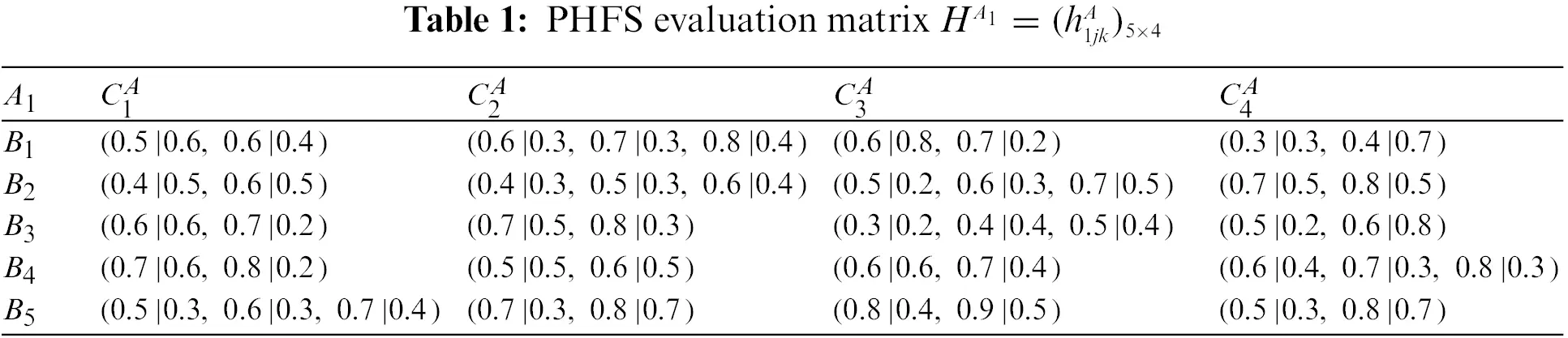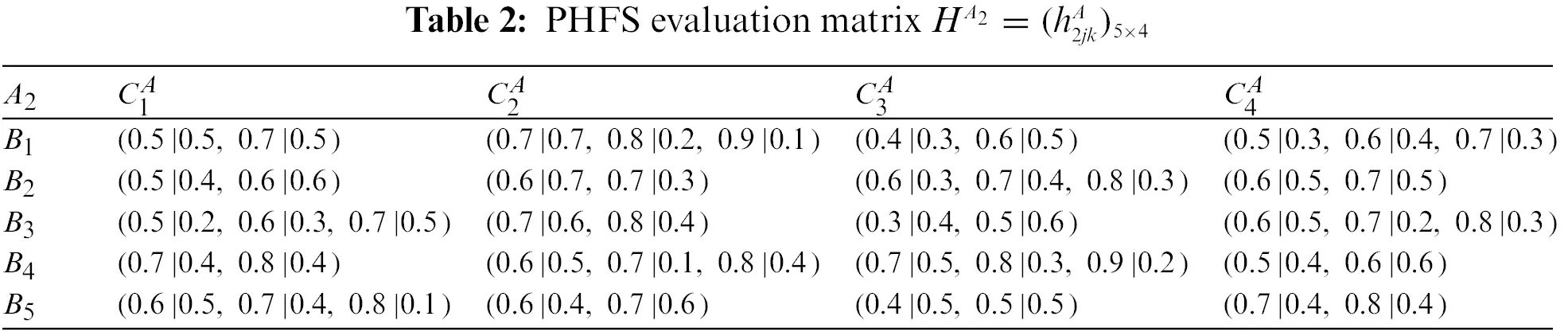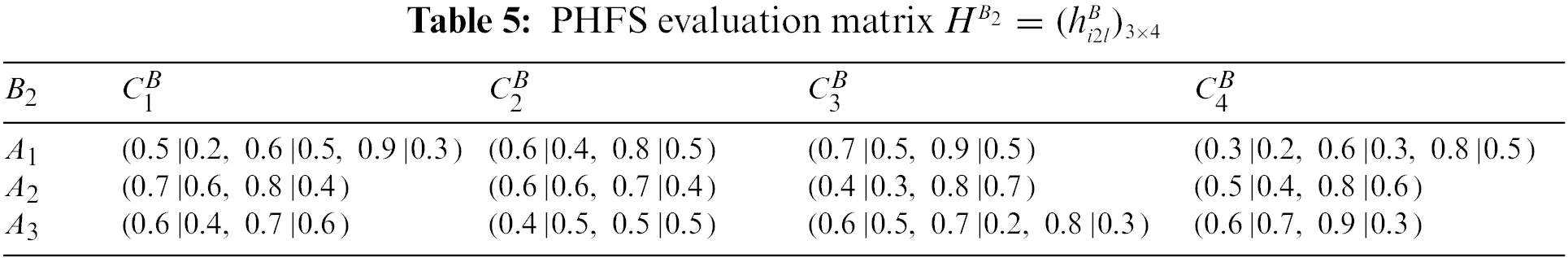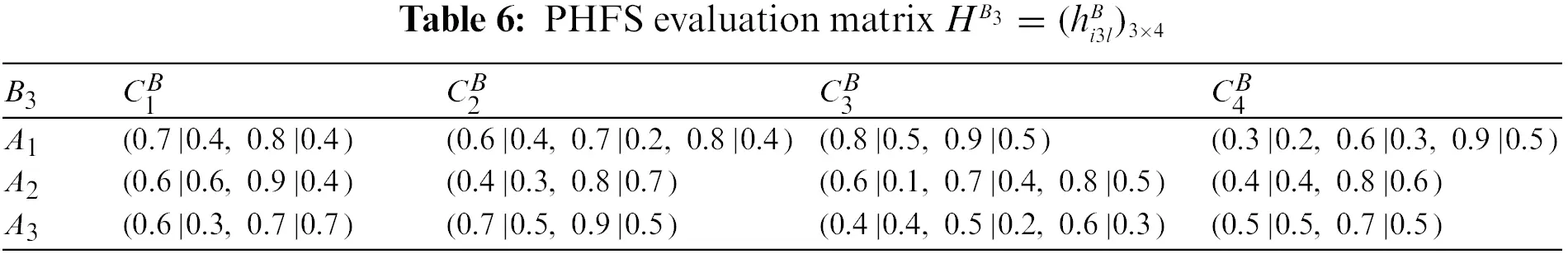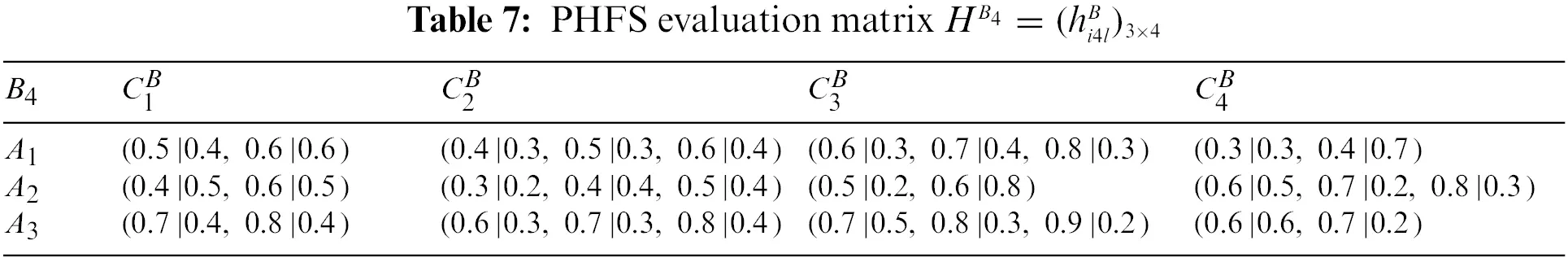Step 1: Construct evaluation matrices.

At the same time, there are differences in the number of membership elements among different PHFEs. Decision-makers are therefore considered to be risk-averse. Based on this, the PHFE, which has a smaller number of membership degrees, is added with the minimal value, and the probability of each additive value is 0. In accordance with the revised method of Definition 1, the PHFE of PHFS evaluation matrix can be revised, and then the PHFS evaluation matrices can be modified and shown in Tables 916.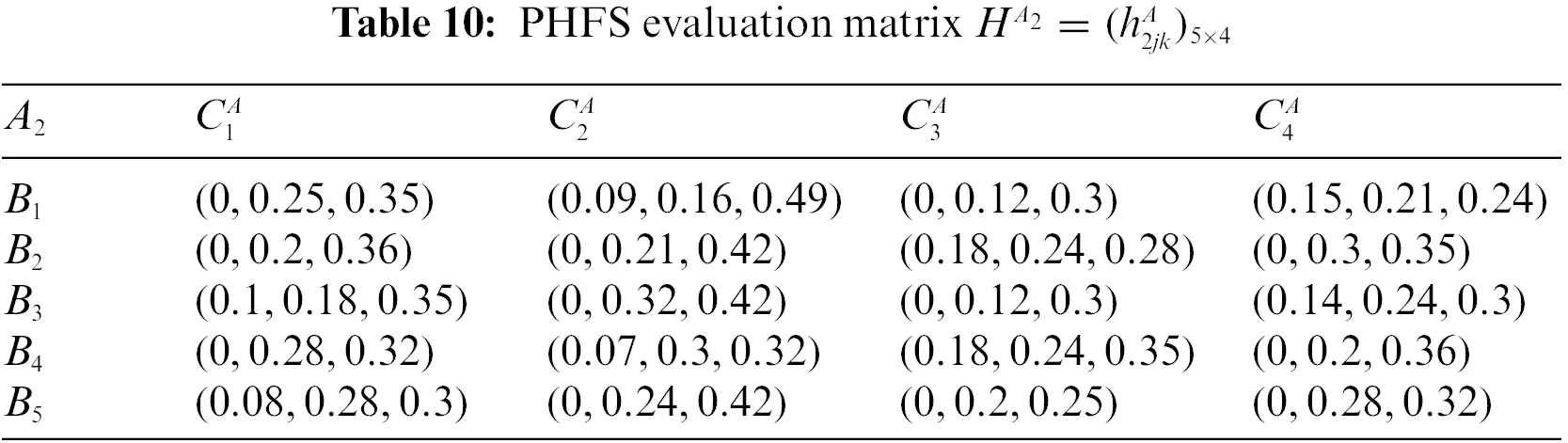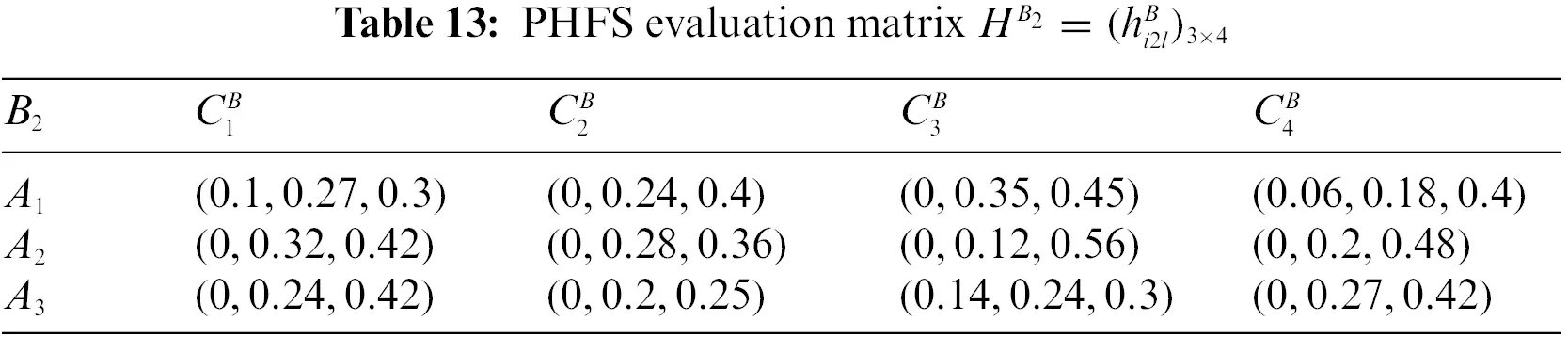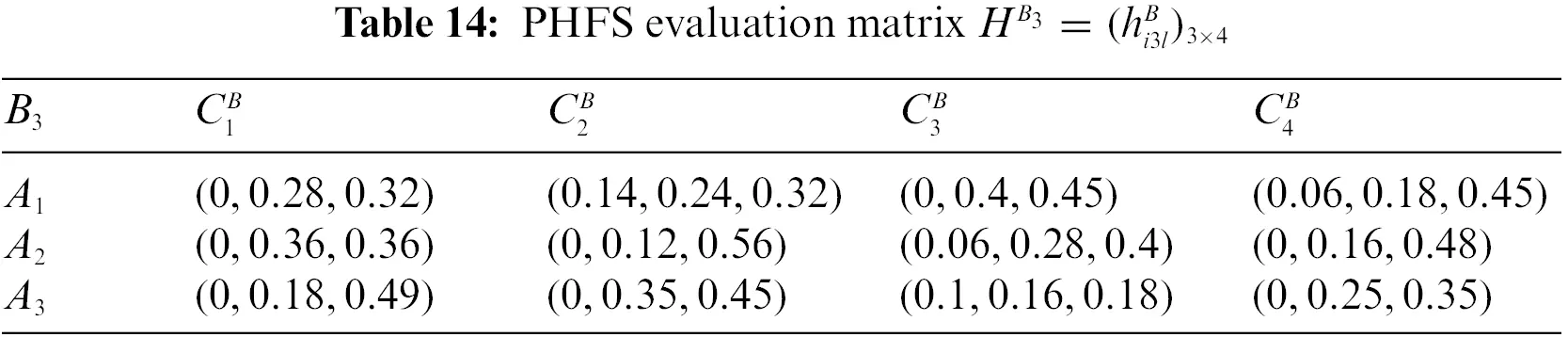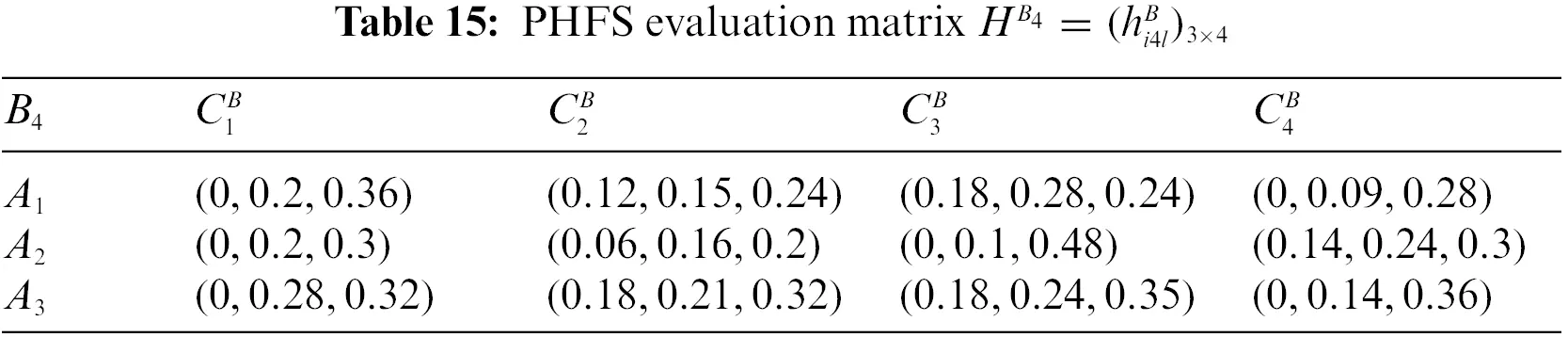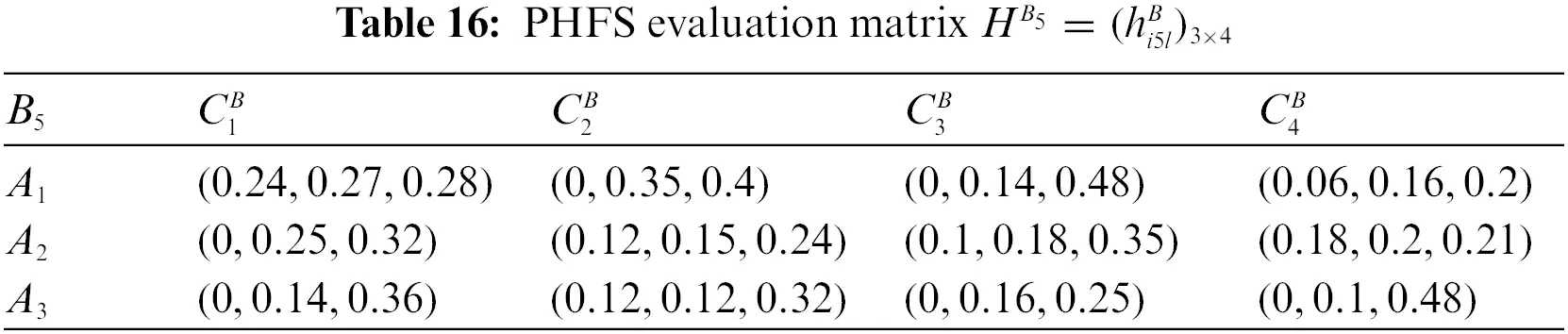Step 2: Compute the attribute weight vector.

Matching is performed by the municipal education bureau/commission using probabilistic hesitant fuzzy evaluation matrices. The information regarding how much each attribute weighs in the evaluation is not provided by two-sided agents. In accordance with the above given steps, the weight information of each attribute can be calculated by Eqs. (12) and (13), that is:

wA1={0.1571,0.2500,0.2500,0.3429},wA2={0.1883,0.2467,0.2971,0.2679},

wA3={0.2709,0.1992,0.2769,0.2530},wB1={0.1914,0.3148,0.2593,0.2436},

wB2={0.2158,0.1655,0.4532,0.1655},wB3={0.1892,0.3297,0.3297,0.1514},

wB4={0.0993,0.2128,0.4255,0.2624},wB5={0.2381,0.2698,0.1958,0.2963}.

Step 3: Comprehensive evaluation matrices.

On this basis, the comprehensive assessment h11A is taken under four attributes CkA(k=1,2,3,4) of primary school A1 to the catering company B1. For instance, we know that

h11A=PHFWA(h111A,h112A,h113A,h114A)=w1A1h111Aw2A1h112Aw3A1h113Aw4A1h114A

Therefore, the comprehensive assessment h11A of A1 to B1 is

h11A=(0.4981|0.0432,0.5239|0.1008,0.5329|0.0108,0.5570|0.0252,0.5329|0.0432,0.5570|0.1008,0.5653|0.0108,0.5877|0.0252,0.5779|0.0576,0.5997|0.1344,0.6072|0.0144,0.6275|0.0336,0.5154|0.0288,0.5403|0.0672,0.5490|0.0072,0.5722|0.0168,0.5490|0.0288,0.5722|0.0672,0.5803|0.0072,0.6019|0.0168,0.5925|0.0384,0.6135|0.0896,0.6208|0.0096,0.6403|0.0224)

The comprehensive PHFS evaluation information of the primary schools can be obtained, as shown in matrix HA. Similarly, the comprehensive PHFS evaluation information of catering companies can also be obtained, as shown in matrix HB.

HA=(h11Ah12Ah13Ah14Ah15Ah21Ah22Ah23Ah24Ah25Ah31Ah32Ah33Ah34Ah35A),HB=(h11Bh12Bh13Bh14Bh15Bh21Bh22Bh23Bh24Bh25Bh31Bh32Bh33Bh34Bh35B)

Step 4: To calculate the distance.

Owing to the number of each PHFE is not equal for hijA, let #hijA be the number, then the positive solution of hijA can be obtained hijA+=(1|1#hijA,1|1#hijA,,1|1#hijA), the negative solution of hijA can be evaluated hijA=(0|1#hijA,0|1#hijA,,0|1#hijA). According to the formula daij+=dH(hijA,hijA+),daij=dH(hijA,hijA), for agent Ai, the distance between the comprehensive evaluation of matching agents and the positive and negative ideal evaluation DA+=(hijA+)3×5,DA=(hijA)3×5 is calculated. Similarly, we can calculate the distance of agent Bj, i.e., dbij+=dH(hijB,hijB+),dbij=dH(hijB,hijB), DB+=(hijB+)3×5,DB=(hijB)3×5.

DA+=(3.01361.70063.05052.69642.87771.91372.69821.73441.84042.85192.93341.81541.74151.76802.8929),

DA=(0.49490.59690.38720.72020.70130.44440.73360.64460.46200.52320.55900.47140.65550.52760.7029),

DB+=(1.68521.70531.75031.71451.75671.85764.54132.95771.83371.01151.80172.80872.79611.85343.0713),

DB=(0.72210.68520.5690.57930.73710.54510.70470.72820.57670.52740.62820.66580.61970.42170.3763).

Step 5: Construct the satisfaction degree matrices.

According to the satisfaction degree formula, the satisfaction degree of the matching agent Ai to the matching agent Bj, and the satisfaction degree of the agent Bj to the agent Ai can be calculated and stated as follows:

SA=(0.85890.74020.88740.78920.80410.81150.78620.72900.79930.8450.83990.79390.72650.77020.8045),

SB=(0.70000.71340.75470.74740.70440.77310.86570.80240.76070.65730.74150.80840.81860.81460.8909).

Step 6–7: Construct the model and solve it.

Based on the satisfaction matrices SA=(φaij)mn and SB=(φbij)mn, the model can be solved by determining the parameters wA and wB. If primary schools and catering companies have the same status, then wA=wB=0.5 and SAB=wASA+wBSB=0.5SA+0.5SB can be obtained by:

SAB=(0.77950.72680.82110.76830.75420.79230.82590.76570.78000.75110.79070.80110.77250.79240.8477).

The optimization model can be solved by utilizing the Lingo 11 software, so that we can get x12=x25=x33=1, and the others xij=0. Therefore, the optimal matching pairs are: (A1,B2),(A2,B5), (A3, B3), which shows that primary school A1 matches the catering company B2, primary school A2 matches the catering company B5, and primary school A3matches the catering company B3.

4.2 Sensitivity Analysis and Discussion

To better illustrate the impact of the weights wA and wB in solving model (16), the paper conducts sensitivity analysis of the model. For simplicity, different values of weights SA=(φaij)mn and SB=(φbij)mn are discussed, and the results are shown in Table 17.The matching objects can express their evaluations more flexibly in PHFS since the decision-maker may hesitate while describing the preference evaluation information. As can be observed, in comparison to the matching objects, information on their assessments is provided in triangular intuitionistic fuzzy sets , hesitant fuzzy numbers  and intuitionistic fuzzy numbers , the method proposed in this paper has more advantages.

Furthermore, the tendency of the object function value Z from cases 1–3 is displayed in Fig. 2. The tendency of the matching scheme xij from cases 1–11 is displayed in Fig. 3. Fig. 4 reveals the tendency of the object function value Z from cases 8–11. Based on Figs. 24 the comprehensive tendency of the object function value Z from cases 1–11 could be acquired, as displayed in Fig. 4.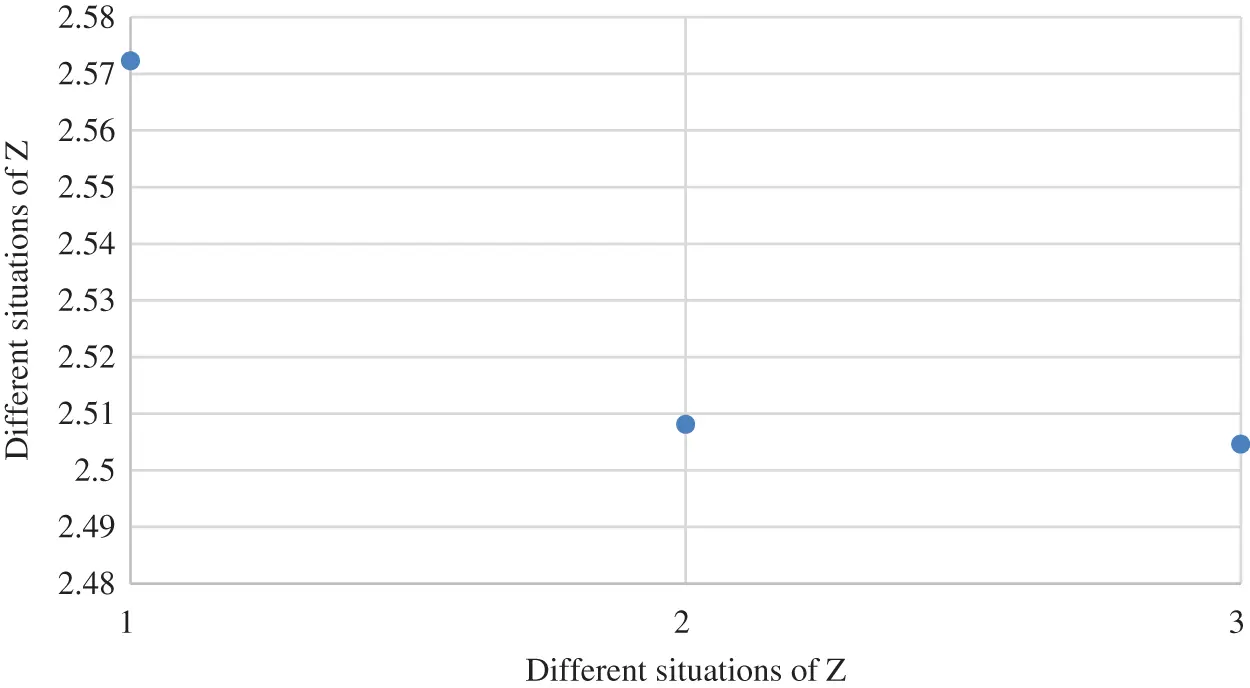Figure 2: The object function value Z from cases 1–3Figure 3: The object function value Z from cases 4–7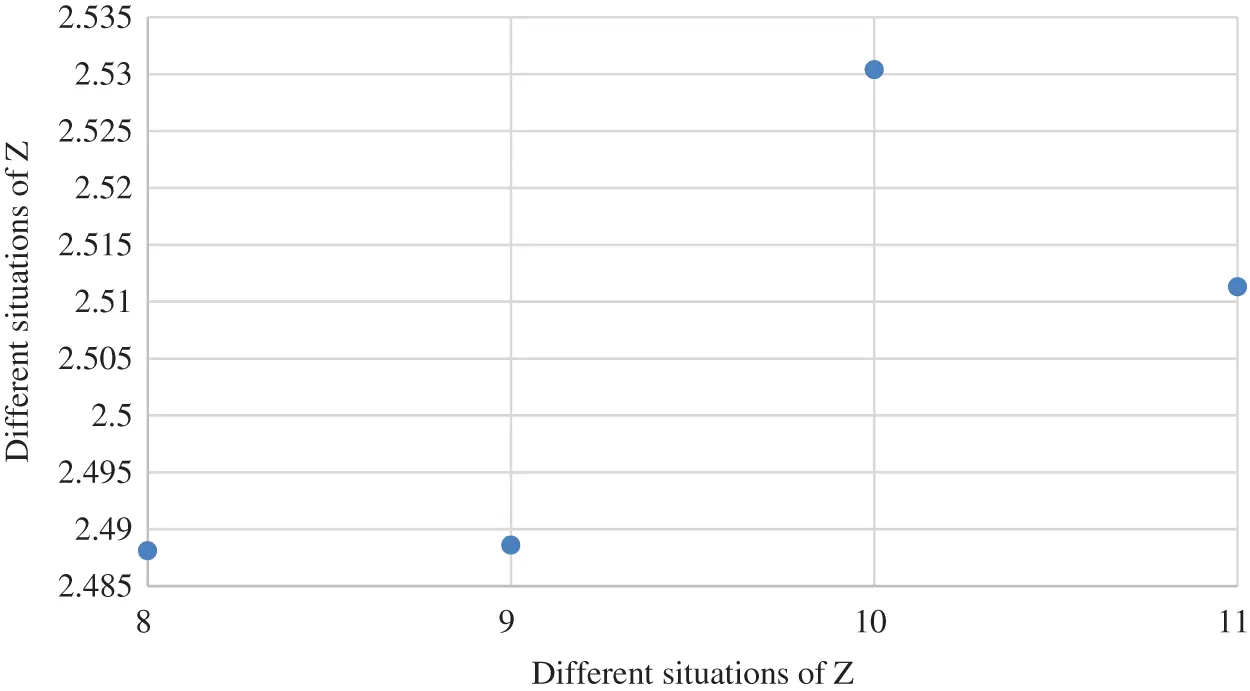Figure 4: The object function value Z from cases 8–11

From Figs. 2 to 4, the paper analyzes the role of two-sided agent weight values on the objective function value, as displayed in Fig. 5. As the weight of the two-sided agent changes from cases 1–2, the object function drops significantly. As the weight changes from cases 2 to 9, the function value gradually decreases until it reaches its lowest point in case 9. However, from cases 9–11, results show that the value of object functioninitially rises and then falls.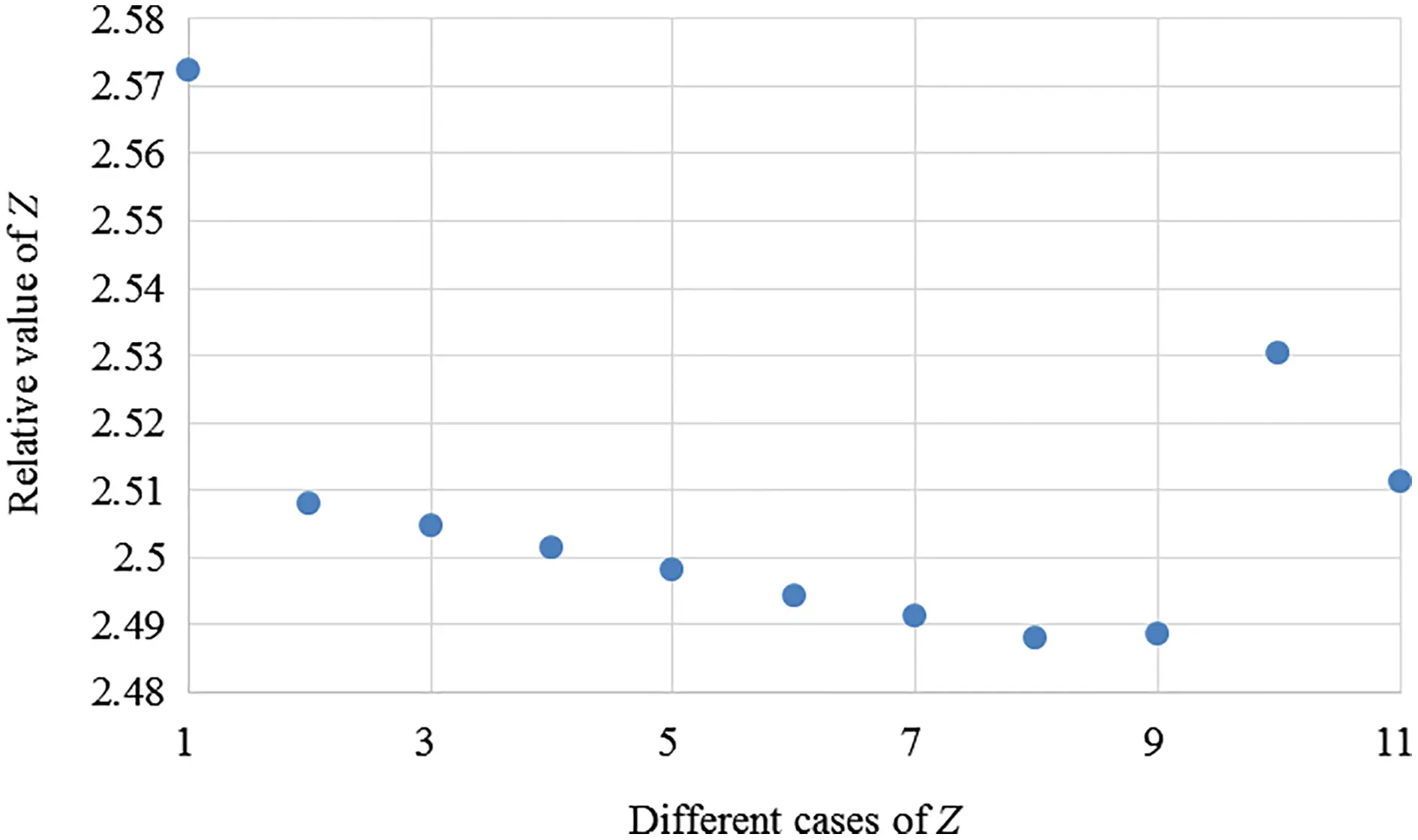Figure 5: The object function value Z from cases 1–11

The tendency of matching scheme xij from cases 1–3 is displayed in Fig. 6. The tendency of matching scheme xij from cases 1–11 is displayed in Fig. 7. The tendency of matching scheme xij from cases 8–11 is revealed in Fig. 8. Based on Figs. 6 to 8, the comprehensive tendency of matching scheme xij from cases 1 to 11 could be acquired, and is displayed in Fig. 9.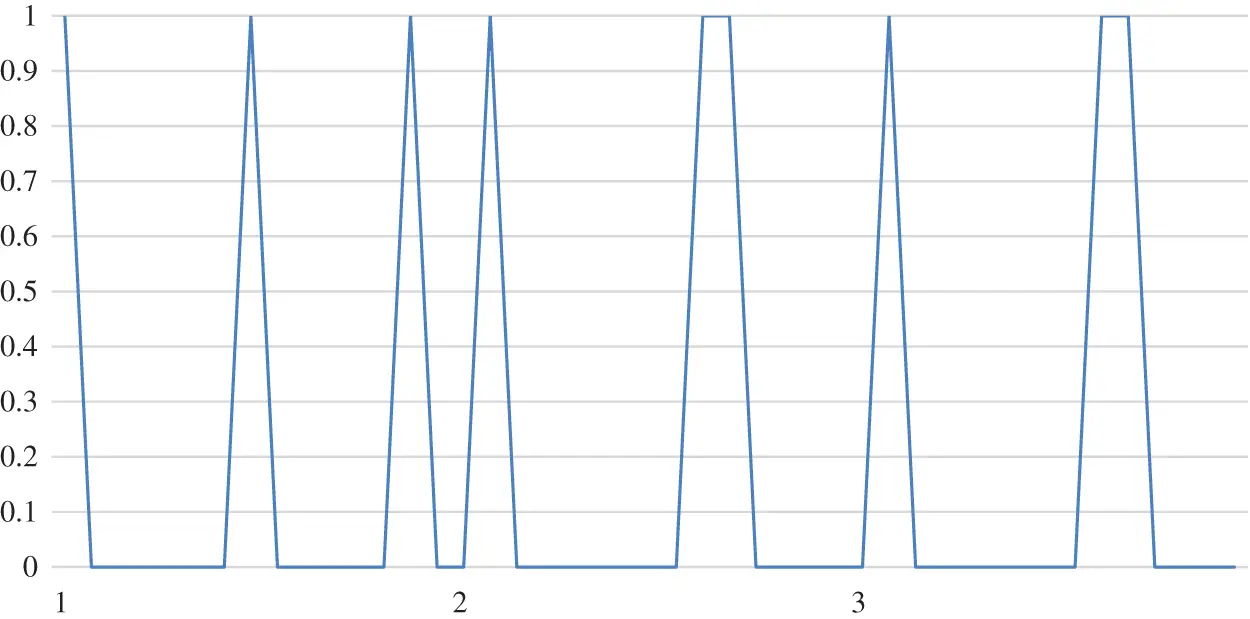Figure 6: The matching scheme xij from cases 1–3Figure 7: The matching scheme xij from cases 4–7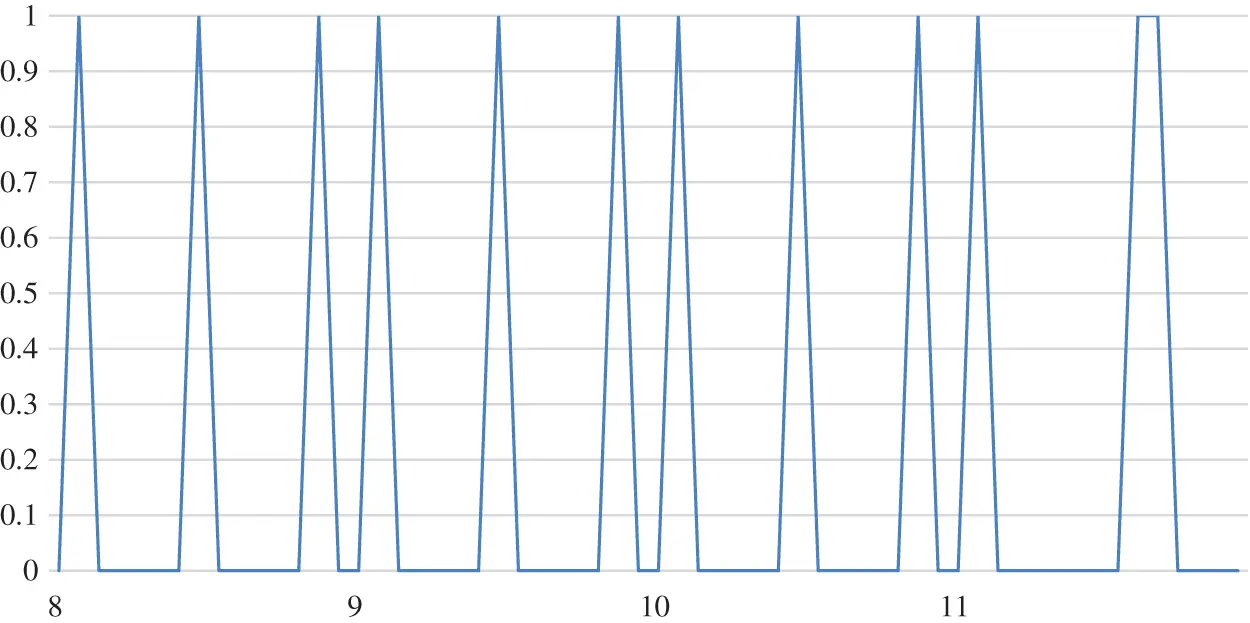Figure 8: The matching scheme xij from cases 8–11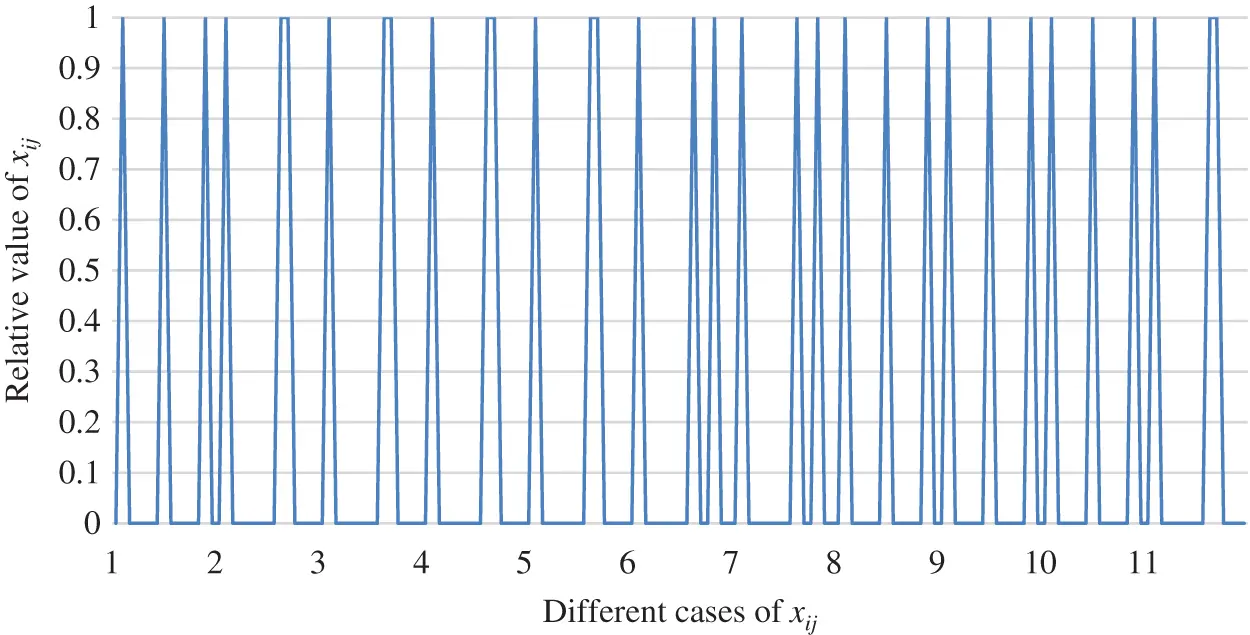Figure 9: The matching scheme xij from cases 1–11

From Figs. 6 to 8, the function of two-sided agent weight values on the matching scheme is examined in the paper. Fig. 9 shows an overall comparison. It can be concluded that the matching results slightly changes with the change in the two-sided agent’s weight. Therefore, it is clear that the weight of the two-sided agent plays no significant role in the change of the matching scheme.

The tendency of the relationship between object function value Z and matching scheme xij from cases 1–3 is displayed in Fig. 10. The tendency of the relationship between object function value Z and matching scheme xij from cases 1–11 is displayed in Fig. 11. The tendency of the relationship between object function value Z and matching scheme xij from cases8–11 is revealed in Fig. 12. The comprehensive tendency of the relationship between object function value Z and matching scheme xij from cases 1–11 could be acquired based on Figs. 10 to 12, as displayed in Fig. 13.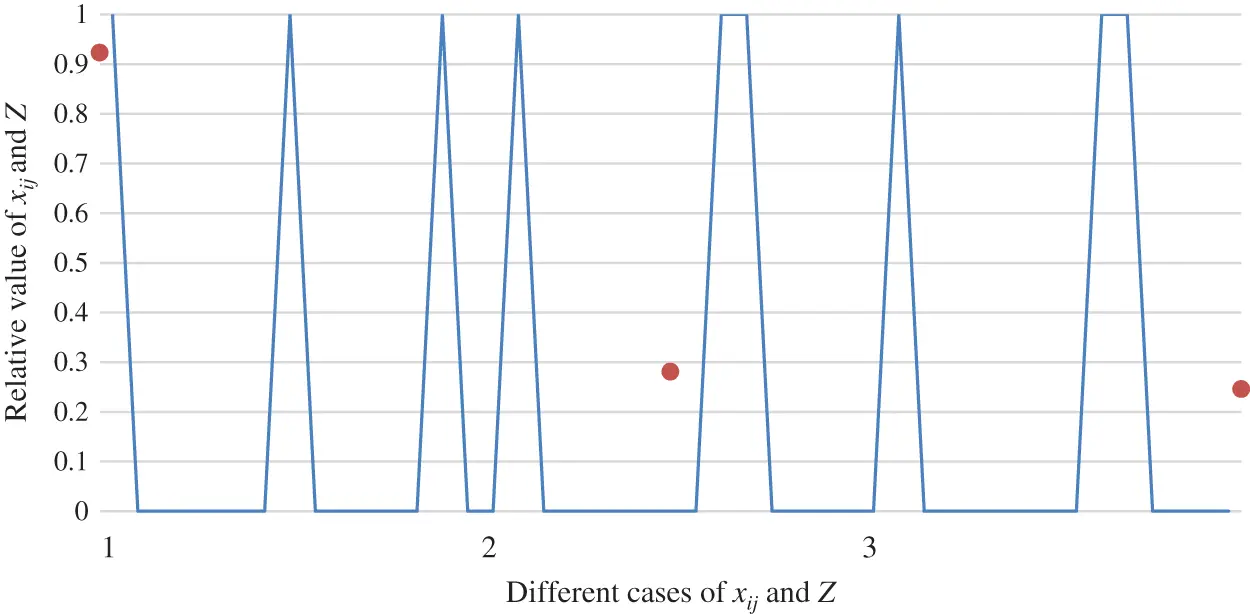Figure 10: The relationship between object function value Z and matching scheme xij from cases 1–3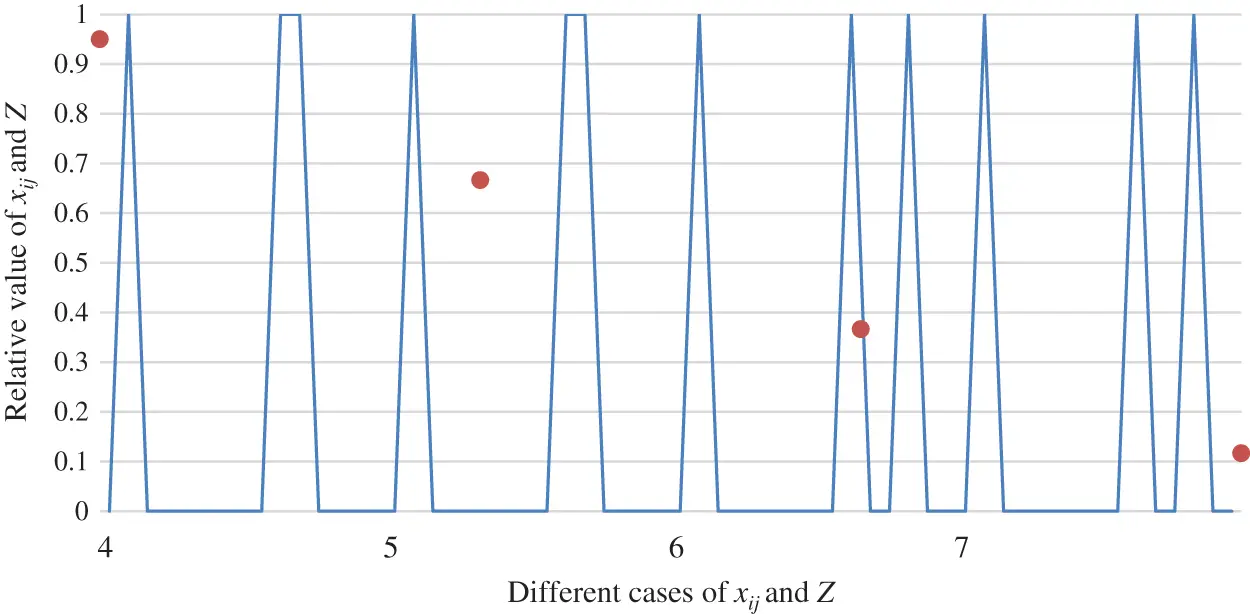Figure 11: The relationship between object function value Z and matching scheme xij from cases 4–7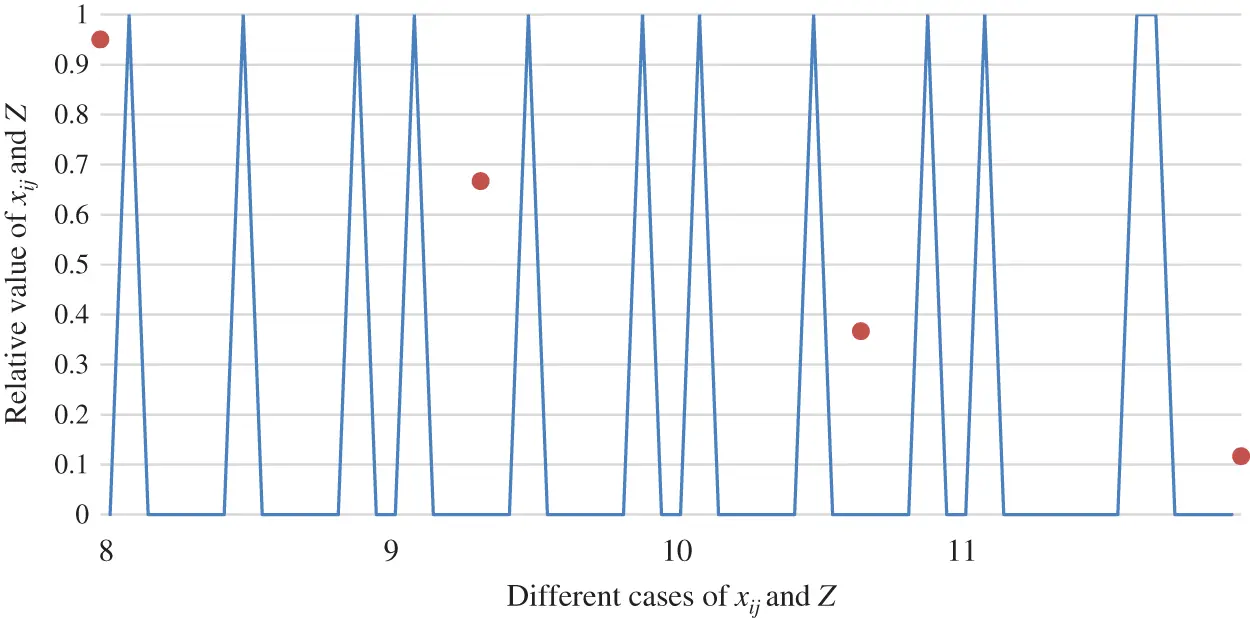Figure 12: The relationship between object function value Z and matching scheme xij from cases 8–11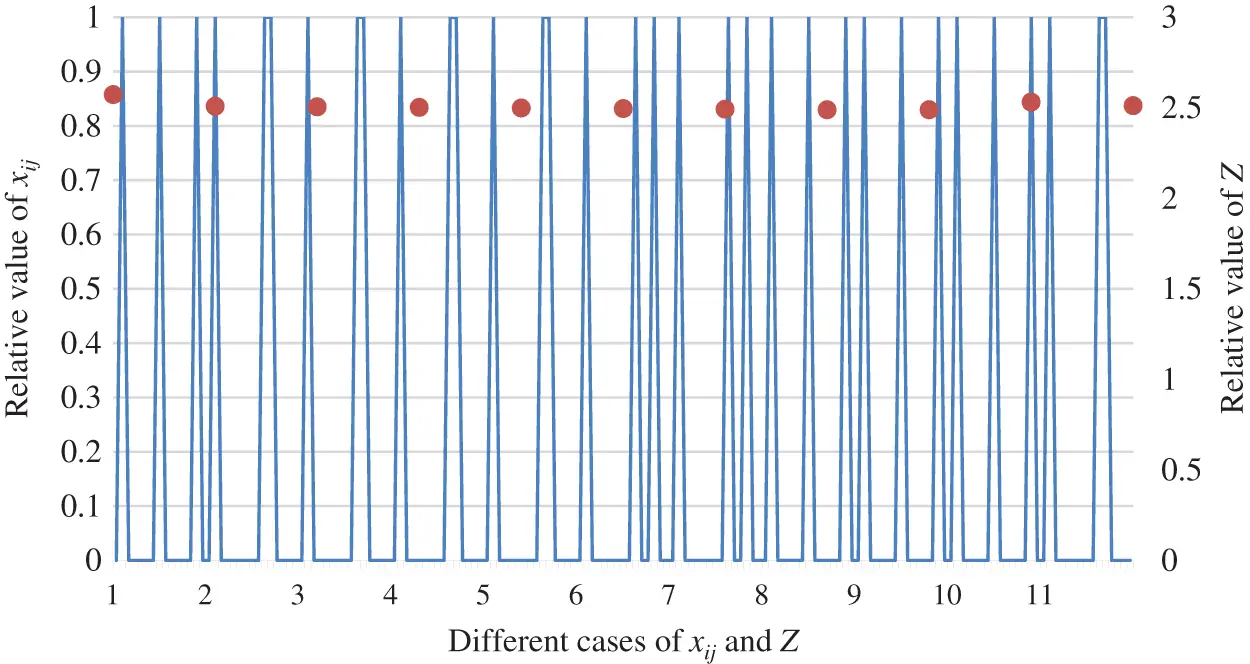Figure 13: The relationship between object function value Z and matching scheme xij from cases 1–11

As shown in Figs. 10 to 12, the paper analyzes the role of two-sided agent weight values on the objection function value and matching scheme. Fig. 13 displays the overall comparison. This leads to the conclusion that a key factor in the change in the value of the objection function is the weight of the two-sided agent. However, the result reveals that the weight of the two-sided agent has no vital role in the change of matching scheme.

Firstly, to better illustrate the advantages of the proposed method for handling fuzzy preference information, the paper compares the proposed method with the one given in . In Reference , the score function is applied to the preference information provided by two-sided agents. Table 18 shows the matching results resulting from two methods. As can be seen in Table 18, there are many differences in the bilateral matching scheme obtained by the above two separate methods. Compared with the method used in reference  to process fuzzy preference information, the distance metric applied in this paper accurately processes the preference evaluation information given by matching agents, thus avoiding the loss and misunderstanding of preference information.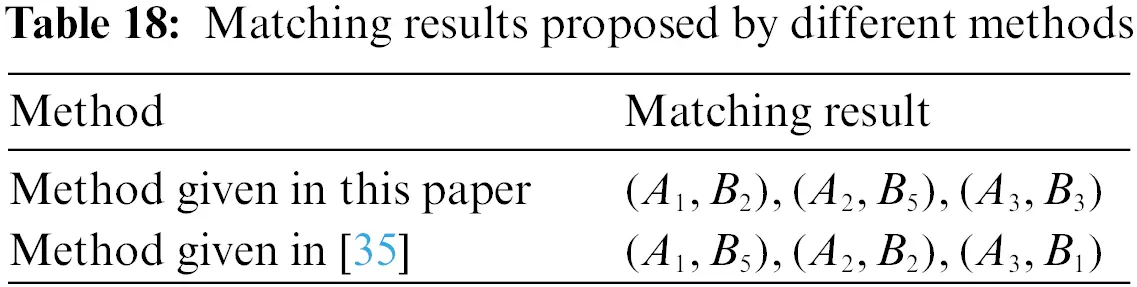Second, in contrast to the traditional TSM research, the methodology used in this paper retains complete information while taking into account hesitant fuzziness and the corresponding probability when evaluating matching objects, making the evaluation more scientific and reasonable, and the results under the probability hesitation fuzzy environment correspond to reality.

Third, multiple attributes are considered, and their weights are calculated by using the maximum deviation method, which can ensure a scientific matching result. Simultaneously, the satisfaction of TSM is acquired by the closeness coefficients of each matching subject, making the entire process more interpretable.

5  Conclusions

In this paper, the TSMDM approach, by considering the attribute of matching objects under probability hesitant fuzzy environment, is developed. The main contributions of this paper are as follows: First, the PHFS is applied to describe the evaluation information provided by matching agents, and the weight vector is incomplete. Second, attribute weight vectors for matching objects are determined using the Hamming distance measures of PHFSs and the extended maximum deviation method based on the unknown weight and probability hesitant fuzzy environment of each matching object. Third, agent satisfaction degrees are gained in accordance with the multi-attribute probability hesitant fuzzy sets comprehensive assessments by using PHFOWA operator. Additionally, the relative closeness coefficient is used to calculate the satisfaction degrees of matched agents. Finally, we build a TSMDM model with the intention of maximizing the overall satisfaction of matching agents, and then the best matching result is determined by solving the constructed TSMDM model with linear weighted method. Overall, the proposed TSMDM approach not only has great potential for application in some real-world TSMDM scenarios, but it could also enhance the theoretical framework for the study of TSMDM problems. The psychology and expectations of the matching subjects when the evaluation information is provided, which is also a future research direction, are not taken into account in this paper. In terms of future research, the future research direction could be presented as follows: (1) For some TSMDM problems, matching objects may provide more evaluation value in hybrid formats, such as the PHFS, probability interval-valued hesitant fuzzy set (PIVHFS) and PHFLTS. In the meanwhile, the matching agents might provide insufficient attribute information. Hence, it could be useful to carry on the discussion of the TSMDM issues with multiple fuzzy information and insufficient attribute values. (2) In the TSMDM process, each matching individual should determine the automatic matching of recommended matching alternatives in the evolutionary environment as a result of the development of artificial intelligence computing systems. In light of these conditions, it could be necessary to develop a novel TSMDM method.

Acknowledgement: The authors would like to thank the editor and anonymous reviewers for their valuable comments and suggestions, thus improving the version of this article.

Funding Statement: This work is supported by the National Natural Science Foundation in China (Yue Qi, Project No. 71861015).

Conflicts of Interest: The authors declare that they have no conflicts of interest regarding the present study.

## References

1. Z. Zhang, X. Kou, W. Yu and Y. Gao, “Consistency improvement for fuzzy preference relations with self-confidence: An application in two-sided matching decision making,” Journal of the Operational Research Society, vol. 72, no. 8, pp. 1914–1927, 202
2. D. Gale and L. S. Shapley, “College admissions and the stability of marriage,” The American Mathematical Monthly, vol. 69, no. 1, pp. 9–15, 196
3. J. Chang, H. Li and B. Z. Sun, “Matching knowledge suppliers and demanders on a digital platform: A novel method,” IEEE Access, vol. 7, pp. 21331–21342, 2019.
4. K. Alexander, “A necessary and sufficient condition for uniqueness consistency in the stable marriage matching problem,” Economics Letters, vol. 178, pp. 63–65, 2019.
5. E. M. Fenoaltea, I. B. Baybusinov, J. Y. Zhao, L. Zhou, and Y. C. Zhang, “The stable marriage problem: An interdisciplinary review from the physicist’s perspective,” The Stable Marriage Problem: An Interdisciplinary Review from the Physicist’s Perspective, vol. 917, pp. 1–79, 2021.
6. A. E. Roth, “The college admissions problem is not equivalent to the marriage problem,” Journal of Economic Theory, vol. 36, no. 2, pp. 277–288, 1985.
7. S. Q. Chen, L. Zhang, H. L. Shi and Y. M. Wang,“Two-sided matching model for assigning volunteer teams to relief tasks in the absence of sufficient information,” Knowledge-Based Systems, vol. 232, no. 28, pp. 1–18, 2021.
8. D. J. Yu and Z. S. Xu, “Intuitionistic fuzzy two-sided matching model and its application to personnel-position matching problems,” Journal of the Operational Research Society, vol. 71, no. 2, pp. 312–321, 2020.
9. Y. M. Miao, R. Du, J. Lin and J. C. Westland, “A Two-sided matching model in the context of B2B export cross-border e-commerce,” Electronic Commerce Research, vol. 19, no. 4, pp. 841–861, 201
10. R. Liang, C. Z. Wu, Z. H. Sheng and X. Y. Wang, “Multi-criterion two-sided matching of public–private partnership infrastructure projects: Criteria and methods,” Sustainability, vol. 10, no. 1178, pp. 1–22, 2018.
11. Y. Liu and K. W. Li, “A Two-sided matching decision method for supply and demand of technological knowledge,” Journal of Knowledge Management, vol. 21, no. 3, pp. 592–606, 2017.
12. T. Wu, M. B. Zhang, X. Tian, S. Y. Wang and G. W. Hua, “Spatial differentiation and network externality in pricing mechanism of online car hailing platform,” International Journal of Production Economics, vol. 219, no. 1, pp. 275–283, 2020.
13. Z. H. Wang, Y. Y. Li, F. Gu, J. F. Guo and X. J. Wu, “Two-sided matching and strategic selection on freight resource sharing platforms,” Physica A: Statistical Mechanics and its Applications, vol. 559, pp. 125014, 2020.
14. Y. Liu, Z. P. Fan and Y. P. Jiang, “Satisfied surgeon-patient matching: A model-based method,” Quality & Quantity, vol. 52, no. 6, pp. 2871–2891, 2018.
15. Y. P. Jiang, Z. P. Fan, H. M. Liang and M. H. Sun, “An optimization approach for existing home seller-buyer matching,” Journal of the Operational Research Society, vol. 70, no. 2, pp. 237–254, 2018.
16. Z. Z. Jiang, W. H. Ip, H. Lau and Z. P. Fan, “Multi-objective optimization matching for one-shot multi-attribute exchanges with quantity discounts in e-brokerage,” Expert Systems with Applications, vol. 38, no. 4, pp. 4169–4180, 2011.
17. D. C. Liang, Y. J. Zhang and W. Cao, “q-rung orthopair fuzzy choquet integral aggregation and its application in heterogeneous multi criteria two-sided matching decision making,” International Journal of Intelligent Systems, vol. 34, pp. 3275–3301, 2019.
18. D. C. Liang, X. He, Z. S. Xu and J. H. Li, “Multi-attribute strict two-sided matching methods with interval-valued preference ordinal information,” Journal of Experimental & Theoretical Artificial Intelligence, vol. 34, no. 4, pp. 545–569, 2021.
19. J. L. Lu and J. Ni,“A Multi-attribute two-sided matching decision method based on multi-granularity probabilistic linguistic MARCOS,” Mathematical Problems in Engineering, vol. 34, no. 3, pp. 1–16, 2022.
20. P. Pu, G. H. Yuan, “Two-sided matching model considering multi-information fusion of stakeholders,” Expert Systems with Applications, vol. 212, pp. 118784, 2023.
21. Z. P. Fan, M. Y. Li and X. Zhang, “Satisfied two-sided matching: A method considering elation and disappointment of agents,” Soft Computing, vol. 22, no. 21, pp. 7227–7241, 2018.
22. X. Chen, J. Wang, H. M. Liang and J. Han, “Hesitant multi-attribute two-sided matching: A perspective based on prospect theory,” Journal of Intelligent and Fuzzy Systems, vol. 36, no. 3, pp. 6343–6358, 2019.
23. J. Qin, S. Fan, H. Liang, C. C. Li and Y. C. Dong, “Two-sided matching decision-making in an incomplete and heterogeneous context: A optimization-based method,” International Journal of Computational Intelligence Systems, vol. 15, no. 23, pp. 1–15, 2022.
24. Y. Lin, Y. M. Wang and K. S. Chin, “An enhanced approach for two-sided matching with 2-tuple linguistic multi-attribute preference,” Soft Computing, vol. 23, no. 17, pp. 7977–7990, 2019.
25. Z. Zhang, J. Gao, Y. Gao and W. Yu, “Two-sided matching decision making with multi-granular hesitant fuzzy linguistic term sets and incomplete criteria weight information,” Expert Systems with Applications, vol. 168, pp. 1–12, 2021.
26. V. Torra,“Hesitant fuzzy sets,” International Journal of Intelligent Systems, vol. 25, no. 6, pp. 529–539, 2010.
27. B. Zhu, “Decision making methods and applications based on preference relations,” Ph.D. Dissertation, University of Southeast University, Nanjing, 2014.
28. X. D. Liu, Z. W. Wang, S. T. Zhang and Y. F. Chen, “Investment decision making along the B & R using critic approach in probabilistic hesitant fuzzy environment,” Journal of Business Economics and Management, vol. 21, no. 6, pp. 1683–1705, 2020.
29. J. Gao, Z. S. Xu and H. C. Liao, “A dynamic reference point method for emergency response under hesitant probabilistic fuzzy environment,” International Journal of Fuzzy Systems, vol. 19, no. 5, pp. 1261–1278, 2017.
30. Q. Y. Ding, Y. M. Wang and M. Goh, “TODIM dynamic emergency decision-making method based on hybrid weighted distance under probabilistic hesitant fuzzy information,” International Journal of Fuzzy Systems, vol. 23, pp. 474–491, 2021.
31. J. P. Liu, C. Huang, J. S. Song, P. C. Du and F. F. Jin, “Group decision making based on the modified probability calculation method and DEA cross-efficiency with probabilistic hesitant fuzzy preference relations,” Computers & Industrial Engineering, vol. 156, pp. 1–15, 2021.
32. S. Zhang, Z. S. Xu and Y. He, “Operations and integrations of probabilistic hesitant fuzzy information in decision making,” Information Fusion, vol. 38, no. 2, pp. 1–11, 2017.
33. J. Ding, Z. S. Xu and N. Zhao, “An interactive approach to probabilistic hesitant fuzzy multi-attribute group decision making with incomplete weight information,” Journal of Intelligent & Fuzzy Systems, vol. 32, pp. 2523–2536, 2017.
34. Z. Su, Z. S. Xu, H. Zhao, Z. N. Hao and B. Chen,“Entropy measures for probabilistic hesitant fuzzy information,” IEEEAccess, vol. 7, pp. 65714–65727, 2019.
35. Q. Yue and L. L. Zhang, “Two-sided matching for hesitant fuzzy numbers in smart intelligent technique transfer,” Mechanical Systems and Signal Processing, vol. 139, pp. 1–11, 2020.
36. M. M. Xia and Z. S. Xu, “Hesitant fuzzy information aggregation in decision-making,” International Journal of Approximate Reasoning, vol. 52, no. 3, pp. 395–407, 2011.
37. F. Y. Zhong and Y. C. Deng, “Audit risk evaluation method based on TOPSIS and choquet fuzzy integral,” American Journal of Industrial and Business Management, vol. 10, pp. 815–823, 2020.
38. Y. M. Wang, “Using the method of maximizing deviations to make decision for multiindices,” Systems Engineering and Electronics, vol. 8, no. 3, pp. 21–26, 1997.
39. Q. Yue, W. C. Zhou and W. Hu, “A new theory of triangular intuitionistic fuzzy sets to solve the two-sided matching problem,” Alexandria Engineering Journal, vol. 63, pp. 57–73, 2022.
40. N. Xiang, Y. J. Dou, B. Y. Xin, K. W. Yang and Y. J. Tan, “High-end equipment: An improved two-sided based S&M matching and a novel pareto refining method considering consistency,” Expert Systems with Applications, vol. 202, pp. 1–12, 2022.

P. Zhao, Q. Yue and Z. Deng, "Two-sided matching decision making with multi-attribute probabilistic hesitant fuzzy sets," Intelligent Automation & Soft Computing, vol. 37, no.1, pp. 849–873, 2023.This work is licensed under a Creative Commons Attribution 4.0 International License , which permits unrestricted use, distribution, and reproduction in any medium, provided the original work is properly cited.

View

Like

## Related articles

• Wei Sun, Hongji Du, Shoubai Nie,...
• Haijiang Liu, Lianwei Cui, Xuebin...
• Xu Han, Huijun Yang, Qiufeng Shen,...
• Wenbo Shi, Jiaqi Wang, Jinxiu...
• Kehong Zhang, Keqiu Li